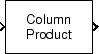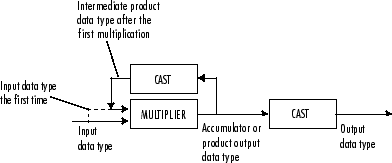# Matrix Product

Multiply matrix elements along rows, columns, or entire input

## Library

Math Functions / Matrices and Linear Algebra / Matrix Operations

`dspmtrx3`

•## Description

The Matrix Product block multiplies the elements of an M-by-N input matrix u along its rows, its columns, or over all its elements.

When the Multiply over parameter is set to `Rows`, the block multiplies across the elements of each row and outputs the resulting M-by-1 matrix. The block treats length-N unoriented vector input as a 1-by-N matrix.

`$\left[\begin{array}{ccc}{u}_{11}& {u}_{12}& {u}_{13}\\ {u}_{21}& {u}_{22}& {u}_{23}\\ {u}_{31}& {u}_{32}& {u}_{33}\end{array}\right]⇒\left[\begin{array}{c}{y}_{1}\\ {y}_{2}\\ {y}_{3}\end{array}\right]=\left[\begin{array}{c}\left(\prod _{j=1}^{3}{u}_{1j}\right)\\ \left(\prod _{j=1}^{3}{u}_{2j}\right)\\ \left(\prod _{j=1}^{3}{u}_{3j}\right)\end{array}\right]$`

When the Multiply over parameter is set to `Columns`, the block multiplies down the elements of each column and outputs the resulting 1-by-N matrix. The block treats length-M unoriented vector input as an M-by-1 matrix.

`$\begin{array}{ccc}\left[\begin{array}{ccc}{u}_{11}& {u}_{12}& {u}_{13}\\ {u}_{21}& {u}_{22}& {u}_{23}\\ {u}_{31}& {u}_{32}& {u}_{33}\end{array}\right]& & \\ ⇓& & \\ \left[\begin{array}{ccc}{y}_{1}& {y}_{2}& {y}_{3}\end{array}\right]& =& \left[\begin{array}{ccc}\left(\prod _{i=1}^{3}{u}_{i1}\right)& \left(\prod _{i=1}^{3}{u}_{i2}\right)& \left(\prod _{i=1}^{3}{u}_{i3}\right)\end{array}\right]\end{array}$`

When the Multiply over parameter is set to ```Entire input```, the block multiplies all the elements of the input together and outputs the resulting scalar.

`$\left[\begin{array}{ccc}{u}_{11}& {u}_{12}& {u}_{13}\\ {u}_{21}& {u}_{22}& {u}_{23}\\ {u}_{31}& {u}_{32}& {u}_{33}\end{array}\right]⇒y=\left(\prod _{i=1}^{3}\prod _{j=1}^{3}{u}_{ij}\right)$`

### Fixed-Point Data Types

The following diagram shows the data types used within the Matrix Product block for fixed-point signals.The output of the multiplier is in the product output data type when at least one of the inputs to the multiplier is real. When both of the inputs to the multiplier are complex, the result of the multiplication is in the accumulator data type. For details on the complex multiplication performed, see Multiplication Data Types. You can set the accumulator, product output, intermediate product, and output data types in the block dialog as discussed in Parameters below.

## Parameters

Main Tab

Multiply over

Indicate whether to multiply together the elements of each row, each column, or the entire input.

Data Types Tab

Note

Floating-point inheritance takes precedence over the data type settings defined on this pane. When inputs are floating point, the block ignores these settings, and all internal data types are floating point.

Rounding mode

Specify the rounding mode for fixed-point operations as one of the following:

• `Floor`

• `Ceiling`

• `Convergent`

• `Nearest`

• `Round`

• `Simplest`

• `Zero`

For more details, see rounding mode.

Saturate on integer overflow

When you select this parameter, the block saturates the result of its fixed-point operation. When you clear this parameter, the block wraps the result of its fixed-point operation. For details on `saturate` and `wrap`, see overflow mode for fixed-point operations.

Intermediate product

Specify the intermediate product data type. As shown in Fixed-Point Data Types, the output of the multiplier is cast to the intermediate product data type before the next element of the input is multiplied into it. You can set it to:

• A rule that inherits a data type, for example, ```Inherit: Same as input```

• An expression that evaluates to a valid data type, for example, `fixdt([],16,0)`

Click the buttonto display the Data Type Assistant, which helps you set the Product output parameter.

Product output

Specify the product output data type. See Fixed-Point Data Types and Multiplication Data Types for illustrations depicting the use of the product output data type in this block. You can set it to:

• A rule that inherits a data type, for example, ```Inherit: Inherit via internal rule```. For more information on this rule, see Inherit via Internal Rule.

• A rule that inherits a data type, for example, ```Inherit: Same as input```.

• An expression that evaluates to a valid data type, for example, `fixdt([],16,0)`

Click the buttonto display the Data Type Assistant, which helps you set the Product output parameter.

Accumulator

Specify the accumulator data type. See Fixed-Point Data Types for illustrations depicting the use of the accumulator data type in this block. You can set this parameter to:

• A rule that inherits a data type, for example, ```Inherit: Inherit via internal rule```. For more information on this rule, see Inherit via Internal Rule.

• A rule that inherits a data type, for example, ```Inherit: Same as input```.

• A rule that inherits a data type, for example, ```Inherit: Same as product output```.

• An expression that evaluates to a valid data type, for example, `fixdt([],16,0)`

Click the buttonto display the Data Type Assistant, which helps you set the Accumulator parameter.

Output

Specify the output data type. See Fixed-Point Data Types for illustrations depicting the use of the output data type in this block. You can set it to:

• A rule that inherits a data type, for example, ```Inherit: Same as product output```

• A rule that inherits a data type, for example, ```Inherit: Same as input```.

• An expression that evaluates to a valid data type, for example, `fixdt([],16,0)`

Click the buttonto display the Data Type Assistant, which helps you set the Output parameter.

Minimum

Specify the minimum value that the block should output. The default value is `[]` (unspecified). Simulink® uses this value to perform:

• Simulation range checking (see Specify Signal Ranges (Simulink))

• Automatic scaling of fixed-point data types

Maximum

Specify the maximum value that the block should output. The default value is `[]` (unspecified). Simulink uses this value to perform:

• Simulation range checking (see Specify Signal Ranges (Simulink))

• Automatic scaling of fixed-point data types

Lock data type settings against changes by the fixed-point tools

Select this parameter to prevent the fixed-point tools from overriding the data types you specify on the block mask.

## Supported Data Types

Port

Supported Data Types

Input

• Double-precision floating point

• Single-precision floating point

• Fixed point

• 8-, 16-, and 32-bit signed integers

• 8-, 16-, and 32-bit unsigned integers

Output

• Double-precision floating point

• Single-precision floating point

• Fixed point

• 8-, 16-, and 32-bit signed integers

• 8-, 16-, and 32-bit unsigned integers

## Extended Capabilities

### Blocks

Introduced before R2006a

## SupportGet trial now